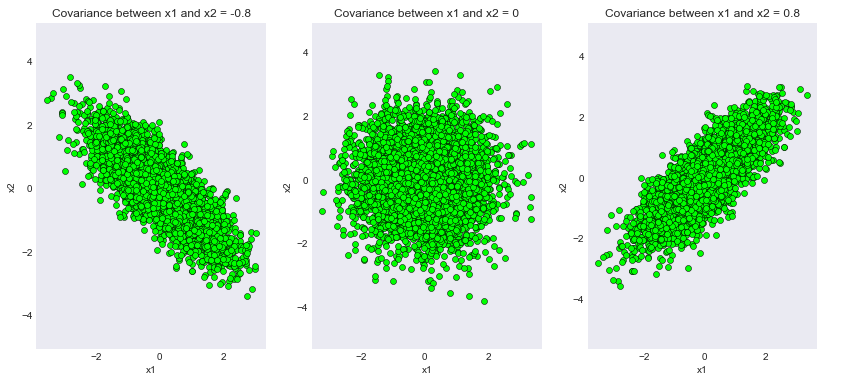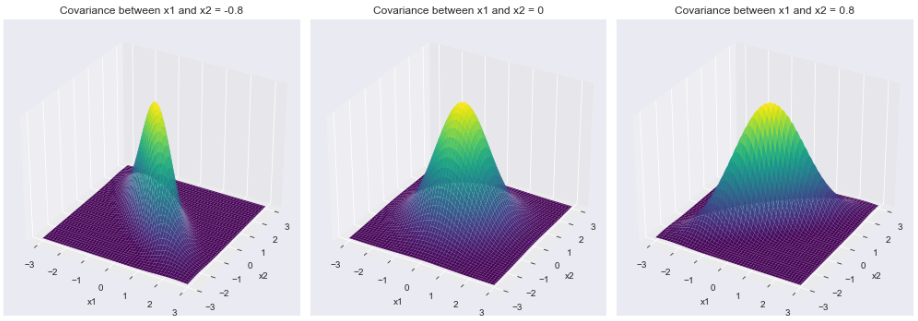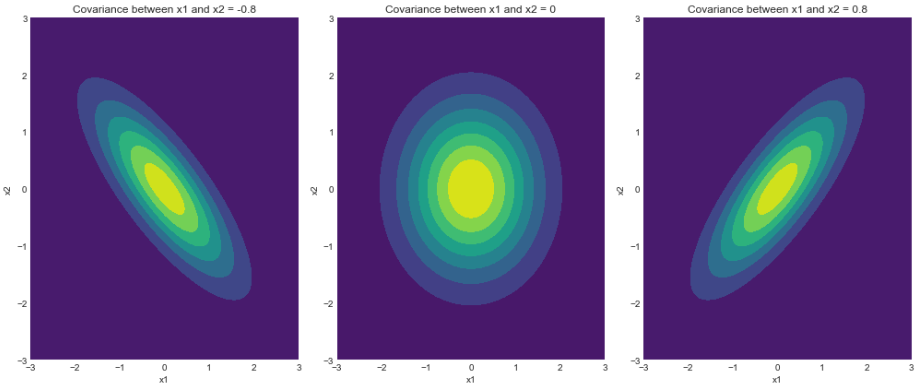Related Articles

# Visualizing the Bivariate Gaussian Distribution in Python

• Difficulty Level : Medium
• Last Updated : 10 Aug, 2021

The Gaussian distribution(or normal distribution) is one of the most fundamental probability distributions in nature. From its occurrence in daily life to its applications in statistical learning techniques, it is one of the most profound mathematical discoveries ever made. This article will ahead towards the multi-dimensional distribution and get an intuitive understanding of the bivariate normal distribution.

The benefit of covering the bivariate distribution is that we can visually see and understand using appropriate geometric plots. Moreover, the same concepts learned through the bivariate distribution can be extended to any number of dimensions. We’ll first briefly cover the theoretical aspects of the distribution and do an exhaustive analysis of the various aspects of it, like the covariance matrix and the density function in Python!

### Probability Density Function(or density function or PDF) of a Bivariate Gaussian distribution

The density function describes the relative likelihood of a random variableat a given sample. If the value is high around a given sample, that means that the random variable will most probably take on that value when sampled at random. Responsible for its characteristic “bell shape”, the density function of a given bivariate Gaussian random variableis mathematically defined as:Whereis any input vectorwhile the symbolsandhave their usual meaning.

Syntax: scipy.stats.multivariate_normal(mean=None, cov=1)

Non-optional Parameters:

• mean: A Numpy array specifyinh the mean of the distribution
• cov: A Numpy array specifying a positive definite covariance matrix
• seed: A random seed for generating reproducible results

Returns: A multivariate normal random variable object scipy.stats._multivariate.multivariate_normal_gen object. Some of the methods of the returned object which are useful for this article are as follows:

• pdf(x): Returns the density function value at the value ‘x’
• rvs(size): Draws ‘size’ number of samples from the generated multivariate Gaussian distribution

### A “visual” view of the covariance matrix

The covariance matrix is perhaps one of the most resourceful components of a bivariate Gaussian distribution. Each element of the covariance matrix defines the covariance between each subsequent pair of random variables. The covariance between two random variablesandis mathematically defined aswheredenotes the expected value of a given random variable. Intuitively speaking, by observing the diagonal elements of the covariance matrix we can easily imagine the contour drawn out by the two Gaussian random variables in 2D. Here’s how:

The values present in the right diagonal represent the joint covariance between two components of the corresponding random variables. If the value is +ve, that means there is positive covariance between the two random variables which means that if we go in a direction whereincreases thenwill increase in that direction also and vice versa. Similarly, if the value is negative that meanswill decrease in the direction of an increase in.

Below is the implementation of the covariance matrix:

In the following code snippets we’ll be generating 3 different Gaussian bivariate distributions with same meanbut different covariance matrices:

1. Covariance matrix with -ve covariance =2. Covariance matrix with 0 covariance =3. Covariance matrix with +ve covariance =## Python

 # Importing the necessary modulesimport numpy as npimport matplotlib.pyplot as pltfrom scipy.stats import multivariate_normal  plt.style.use('seaborn-dark')plt.rcParams['figure.figsize']=14,6 # Initializing the random seedrandom_seed=1000 # List containing the variance# covariance valuescov_val = [-0.8, 0, 0.8] # Setting mean of the distributino to# be at (0,0)mean = np.array([0,0]) # Iterating over different covariance# valuesfor idx, val in enumerate(cov_val):    plt.subplot(1,3,idx+1)         # Initializing the covariance matrix    cov = np.array([[1, val], [val, 1]])         # Generating a Gaussian bivariate distribution    # with given mean and covariance matrix    distr = multivariate_normal(cov = cov, mean = mean,                                seed = random_seed)         # Generating 5000 samples out of the    # distribution    data = distr.rvs(size = 5000)         # Plotting the generated samples    plt.plot(data[:,0],data[:,1], 'o', c='lime',             markeredgewidth = 0.5,             markeredgecolor = 'black')    plt.title(f'Covariance between x1 and x2 = {val}')    plt.xlabel('x1')    plt.ylabel('x2')    plt.axis('equal')     plt.show()

Output:Samples generated for different covariance matrices

We can see that the code’s output has successfully met our theoretical proofs! Note that the value 0.8 was taken just for convenience purposes. The reader can play around with different magnitudes of covariance and expect consistent results.

#### 3D view of the probability density function:

Now we can move over to one of the most interesting and characteristic aspects of the bivariate Gaussian distribution, the density function! The density function is responsible for the characteristic bell shape of the distribution.

## Python

 # Importing the necessary modulesimport numpy as npimport matplotlib.pyplot as pltfrom scipy.stats import multivariate_normal  plt.style.use('seaborn-dark')plt.rcParams['figure.figsize']=14,6fig = plt.figure() # Initializing the random seedrandom_seed=1000 # List containing the variance# covariance valuescov_val = [-0.8, 0, 0.8] # Setting mean of the distributino# to be at (0,0)mean = np.array([0,0]) # Storing density function values for# further analysispdf_list = [] # Iterating over different covariance valuesfor idx, val in enumerate(cov_val):         # Initializing the covariance matrix    cov = np.array([[1, val], [val, 1]])         # Generating a Gaussian bivariate distribution    # with given mean and covariance matrix    distr = multivariate_normal(cov = cov, mean = mean,                                seed = random_seed)         # Generating a meshgrid complacent with    # the 3-sigma boundary    mean_1, mean_2 = mean, mean    sigma_1, sigma_2 = cov[0,0], cov[1,1]         x = np.linspace(-3*sigma_1, 3*sigma_1, num=100)    y = np.linspace(-3*sigma_2, 3*sigma_2, num=100)    X, Y = np.meshgrid(x,y)         # Generating the density function    # for each point in the meshgrid    pdf = np.zeros(X.shape)    for i in range(X.shape):        for j in range(X.shape):            pdf[i,j] = distr.pdf([X[i,j], Y[i,j]])         # Plotting the density function values    key = 131+idx    ax = fig.add_subplot(key, projection = '3d')    ax.plot_surface(X, Y, pdf, cmap = 'viridis')    plt.xlabel("x1")    plt.ylabel("x2")    plt.title(f'Covariance between x1 and x2 = {val}')    pdf_list.append(pdf)    ax.axes.zaxis.set_ticks([]) plt.tight_layout()plt.show() # Plotting contour plotsfor idx, val in enumerate(pdf_list):    plt.subplot(1,3,idx+1)    plt.contourf(X, Y, val, cmap='viridis')    plt.xlabel("x1")    plt.ylabel("x2")    plt.title(f'Covariance between x1 and x2 = {cov_val[idx]}')plt.tight_layout()plt.show()

Output:

1) Plot of the density functionDensity functions corresponding to different covariance matrices

2) Plot of contoursContours of the density functions

As we can see, the density function’s contours exactly match the samples drawn by us in the previous section. Note that the 3 sigma boundary(concluded from the 68-95-99.7 rule) ensures maximum sample coverage for the defined distribution. As mentioned earlier, the reader can play around with different boundaries and expect consistent results.

### Conclusion

We understood the various intricacies behind the Gaussian bivariate distribution through a series of plots and verified the theoretical results with the practical findings using Python. The reader is encouraged to play around with the code snippets for gaining a much more profound intuition about this magical distribution!

Attention geek! Strengthen your foundations with the Python Programming Foundation Course and learn the basics.

To begin with, your interview preparations Enhance your Data Structures concepts with the Python DS Course. And to begin with your Machine Learning Journey, join the Machine Learning – Basic Level Course

My Personal Notes arrow_drop_up